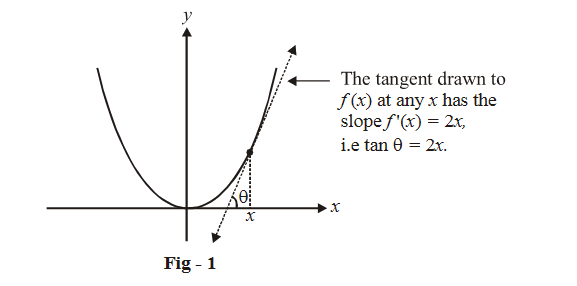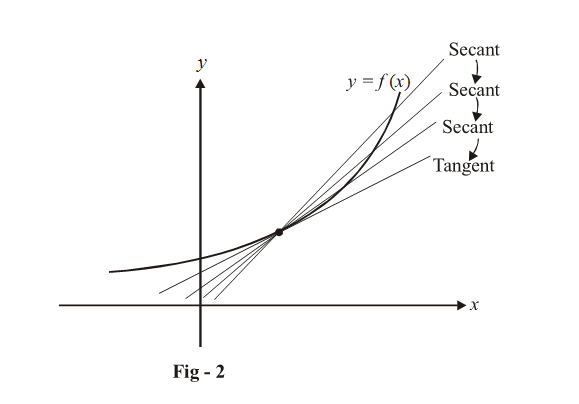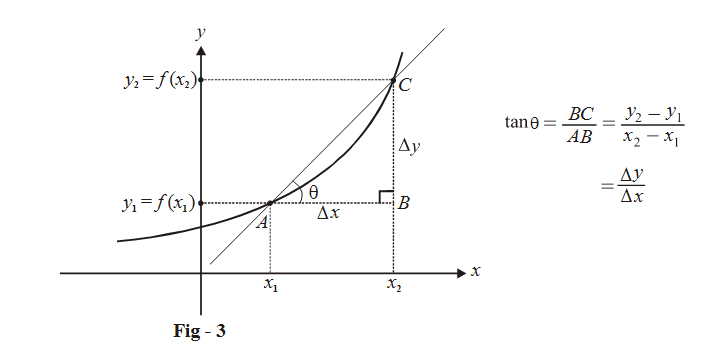# Introduction To Differentiation

Go back to  'LCD'

Now that we have seen the concepts of continuity and differentiability, we will start developing applications of these concepts. These applications include differentiation (developing techniques and formulae to differentiate a given function), maxima and minima, drawing tangents and normals (to some given curve at some given point), studying monotonicity of functions, and so on. In this chapter, we will study differentiation which is just an extension of the differentiability concept (in fact, we already have studied almost everything pertaining to differentiation). Our purpose here is to find out the derivatives of some standard functions by first principles, and then use these results whenever we want to differentiate any arbitrary given function.

In the previous chapter, we saw in great detail the meaning of evaluating the derivative (differentiating) of a given function at any given point.

We summarize that discussion briefly here:

If $$f(x)$$ is a differentiable function for a given $$x$$, this means that we can draw a unique tangent to $$f(x)$$ for that given $$x$$. The slope of this unique tangent is called the derivative of $$f(x)$$ for that given $$x$$. The process of finding the derivative is known as differentiation .

For example, for $$f\left( x \right) = {x^2},$$ the derivative at any given $$x$$ has the value $$2x$$ (we evaluated this by first principles in the previous chapter.) This means that the slope of the tangent drawn to $$f\left( x \right)$$ at any given $$x$$has the numerical value $$2x$$.

Equivalently stated, we can differentiate $$f\left( x \right) = {x^2}$$ to get $$f'\left( x \right) = 2x$$ .

$$\left\{ {f'\left( x \right){\rm{\;represents\;the\;derivative\;of\;}}\,f(x)} \right\}.$$Recall that we can differentiate a function at a given point only if the LHD and RHD at that point have equal values. If they do, then

$f'\left( x \right) = \frac{{d\left( {f\left( x \right)} \right)}}{{dx}} = \mathop {\lim }\limits_{h \to 0} \frac{{f\left( {x + h} \right) - f\left( x \right)}}{h} = \mathop {\lim }\limits_{h \to 0} \frac{{f\left( {x - h} \right) - f\left( x \right)}}{{ - h}}$

The notation that we use to signify the derivative of $$y = f\left( x \right)$$ is either $$f'\left( x \right)\left( {{\rm{or}}\,y'} \right)$$ or $$\frac{{d\,f\left( x \right)}}{{dx}}\left( {{\rm{or}}\frac{{dy}}{{dx}}} \right)$$

We need to understand here the significance of the notation \begin{align}\frac{{dy}}{{dx}}\end{align} (the derivative of $$y$$ with respect to the variable $$x$$)

Recall that to evaluate the derivative (slope of the tangent) of $$y = f(x)$$ at a given point, we first drew a secant passing through that point and then let that secant tend to a tangent as follows:The slope of any secant can be written easily:The slope is obvious from the figure:

\begin{align}\tan \theta = \frac{{BC}}{{AB}} = \frac{{{y_2} - {y_1}}}{{{x_2} - {x_1}}} = \frac{{\Delta y}}{{\Delta x}}\end{align}

Now, to evaluate the derivative at $$x_1$$, we need to make the secant AC tend to a tangent at A by letting$$x_2$$ approach $${x_1}\left( {{x_2} \to {x_1}} \right)$$ or equivalently, by letting $$\Delta x \to 0.$$

As $$\Delta x$$ becomes an infinitesmally small quantity (approaches 0), the corresponding $$\Delta y$$ will also become infinitesmally small (will approach 0), but the ratio \begin{align}\frac{{\Delta y}}{{\Delta x}}\end{align} will become an increasingly accurate representation of the slope of the tangent at A.

An infinitesmally small change in the x value is represented by $$dx$$ instead of $$\Delta x.$$

Similarly, an infinitesmally small change in the y value would be represented by $$dy$$ instead of $$\Delta y.$$

Therefore,

\begin{align}\mathop {\lim }\limits_{{x_2} \to {x_1}} \frac{{\Delta y}}{{\Delta x}} = \mathop {\lim }\limits_{\Delta x \to 0} \frac{{\Delta y}}{{\Delta x}} = \frac{{dy}}{{dx}} = y'\end{align}

You should now be clear about the notation \begin{align}\frac{{dy}}{{dx}}\end{align} . We will use \begin{align}\frac{{dy}}{{dx}},\frac{{d\,f\left( x \right)}}{{dx}},\,f'\left( x \right)\,\,{\rm{or}}\,\,y'\end{align} interchangeably to represent the derivative of $$y = f\left( x \right)$$ at any given $$x$$.

Note: From now on you should always keep in mind that d (variable) represents an infinitesmally small change in the variable value while $$\Delta$$ (variable) represents a finite change in the variable value.